+ +

# Astigmatism and graph "double plot."

The representation of theastigmatism on a graph "double plot" allows to use the vector calculation without performing arithmetic on the angles (corresponding to the axis of astigmatism)

# Graph double Stud

It provides a representation of convenient to represent geometric way of operations performed on astigmatism: additions, average, subtraction, etc.

## Conditions:

It is necessary to use the same sign (positive or negative) for the vector calculations of astigmatism.

Representation in mode "double plot" does not allow to calculate changes in the spherical power (in the sense of the sphero-cylindrical refractive) resulting from the addition of the spires of the astigmatism.

## Principle:

The mode "double plot" is a space plan with a particular trigonometric reference in which it represents the astigmatism as a vector (polar coordinates): the length of the arrow is the magnitude, and the angle axis of astigmatism.

In this space, the angles have equal Double their value in such degree as represented in a classical reference (0 ° / 360 °). Thus, in the representation mode double plot, the angular frequency is 0 ° / 180 °, which is consistent with the astigmatism.

When we represent a set of data (ex: respective astigmatismes of a group of eyes), it is better to use a simple point, which one can easily deduce the polar coordinates.

That's reverse astigmatismes (or indirect, "the rule against" = ATR) will be represented by arrows (or points) located in the dial 'right', so that the direct astigmatismes ("with the rule" = WTR) its represented by arrows or points located in the 'left' dial Oblique directions fit into the top and bottom dials.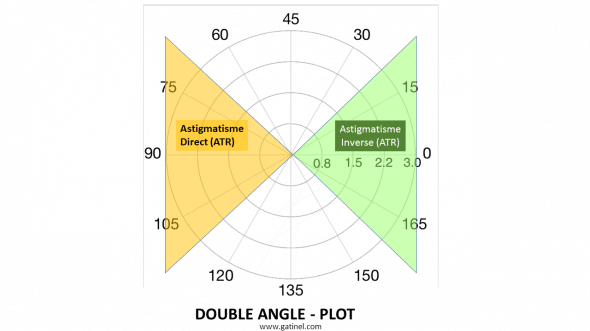The graph can be divided into quadrants, where points or vectors corresponding to the direction of astigmatism will be screened.

An ocular astigmatism can be corrected with a device that generates an astigmatism of same magnitude but opposite direction (90 ° in the case of the axis of astigmatism). In this example, a reverse astigmatism (red arrow) is "corrected" by a reverse astigmatism from opposite direction (blue arrow). In mode 'double plot", the 90 ° angle corresponds to a flat angle (180 °):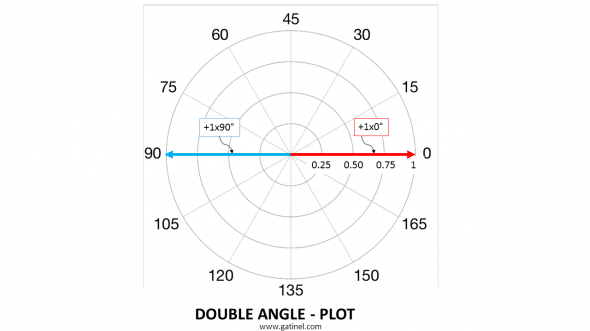A reverse astigmatism (red arrow) and direct (blue arrow) of the same magnitude but opposite direction (90 ° corresponding to 180 ° on the graph representation) neutralize perfectly.

In the following example, we can represent the effect of the addition of two astigmatismes which is opposite, the other direct: this modeling may be that of a corneal astigmatism (1 d) corrected with a toric implant (1 d) which the axis of astigmatism is slightly offset (15 °) in the eye, with respect to the direction expected (90 °):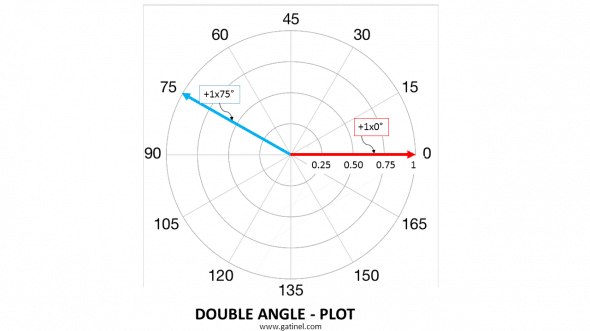Reverse astigmatism (red arrow) corresponds to a corneal astigmatism, astigmatism modeled by the blue arrow corresponds to that induced by the implant which axis is deviated from 15 ° to its 'ideal' direction (90 °).

Astigmatism resulting from this addition is deducted by the addition of the spires of vectors:

In this example, we can use vector calculation to estimate the value of surgically induced astigmatism (Surgically induced astigmatism: SIA) from the vectors modeling pre-operative corneal astigmatism (+ 3 × 0 °) and residual astigmatism after establishing a toric implant 3D cylinder power. The calculation allows to establish the direction of astigmatism correction is offset 5 ° with respect to the direction expected (0 °).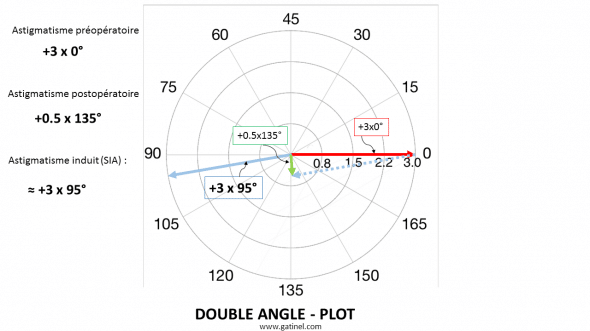An error of (5) just to the induction of a significant residual astigmatism for a toric implant correcting 3 D of corneal astigmatism.

Post surgical astigmatism is the result of the combined effect of the correction device (ex: toric implant) and other parameters like the surgical incision. In this representation, the successive addition of the arrows of astigmatism to calculate final astigmatism. The effect of incision is, in this example, beneficial.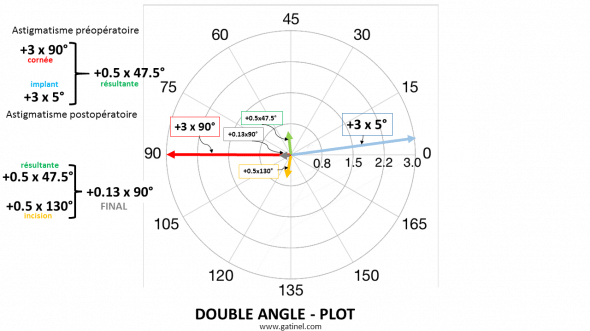Calculation of the stacks of the incision and the o-ring implant opposite of initial astigmatism (corneal).

### One response to "astigmatism and graph" double plot "

1.Dr. Damien Gatinel says:

Zero degrees is always located "right" on the graph so the nose for the right eye and head for the left eye.